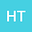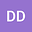Inverse coefficient problem for a time - fractional wave equation with initial - boundary and integral type overdetermination conditions
••D. K. Durdiev
Bukhara branch of the institute of Mathematics named after VI Romanovskiy at the Academy of sciences of the Republic of Uzbekistan
Author Profile## Abstract

This paper considers the inverse problem of determining the time-dependent coefficient in the time-fractional diffusion-wave equation. In this case, an initial boundary value problem was set for the fractional diffusion-wave equation, and an additional condition was given for the inverse problem of determining the coefficient from this equation. First of all, it was considered the initial boundary value problem. By the Fourier method, this problem is reduced to equivalent integral equations. Then, using the Mittag-Leffler function and the generalized singular Gronwall inequality, we get apriori estimate for solution via unknown coefficient which we will need to study of the inverse problem. The inverse problem is reduced to the equivalent integral of equation of Volterra type. The principle of contracted mapping is used to solve this equation. Local existence and global uniqueness results are proved. The stability estimate is also obtained.
22 Nov 2022Submitted to Mathematical Methods in the Applied Sciences
22 Nov 2022Assigned to Editor
22 Nov 2022Submission Checks Completed
28 Nov 2022Review(s) Completed, Editorial Evaluation Pending
04 Dec 2022Reviewer(s) Assigned
23 Feb 2023Editorial Decision: Revise Major